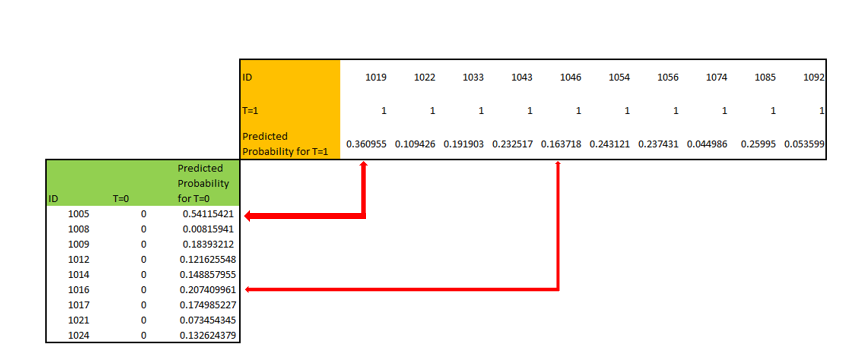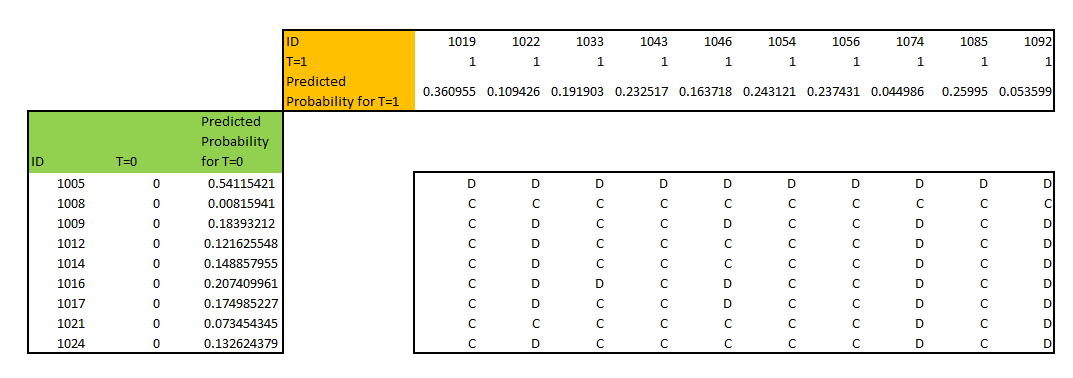## Model Performance Statistics – Concordance: Calculation Steps

By: Ram on Jun 19, 2020

Some of the commonly used  Model Performance Statistics for Binary Predictive Models are:

• Confusion Matrix
• ROC Chart
• Concordance %
• Gini
• KS
• Lift Chart
• Gain Table and Chart

In this blog, we will show the steps involved in calculating concordance %.

In a binary predictive modeling scenario, we have two important variables:  Observed Target Variable and Predicted Outcome Variable.  For example, in a credit risk default scenario, we have created a target variable that takes value 1 (if a customer has defaulted) and 0. This is our observed target variable. Based on the predictive model especially when a logistic regression technique is used, we get predicted probabilities which are the Predicted Outcome Variable.

Compare Observed Target Variable values and Predicted Outcome Variable values to assess the efficacy of the predictive model.

In concordance, predicted probabilities of Observed Target variable value 1 is compared against the predicted probabilities of Observed Target Variable value 0. If predicted probabilities value for observation with Observed Target variable 1 is higher than the predicted probabilities value for observation with Observed Target variable 0, then it is concordant otherwise discordance. When the predicted probabilities for observed target variable value 1 and 0 is equal, it is called tied.

### Steps:

1. Columns – ID variable, Observed Target variable and Predicted Probabilities (based on a model)
2. Find pairs – for comparison, we need to find all probable pairs. Candidates with Observed Target Variable 1 are compared with the candidates with Observed Target variable value 0. In this example, Target variable 0 has 9 observations and target variable 1 has 10 observations. So, all comparison pairs are 9*10=90.
3. We can create a comparison matrix structured as follow.4. Categorize pair into Concordant, Discordant or Tied based on Predicted Probabilities.
1. Concordant: If Predicted Probability for Observed Target variable value 1 is more than that of target variable value 0
2. Discordant: If Predicted Probability for Observed Target variable value 0 is more than that of target variable value 1
3. Tied: If Predicted Probability for Observed Target variable value 0 is equal to that of target variable value 15. Calculate % Concordance

# of Pairs: 9*10=90% Concordance:  56/90 => 62%% Discordance:    34/90 => 38%% Tied: 0/90 => 0%

Tags
Most PopularJun 18, 2020Jul 23, 2020Jun 19, 2020Jun 19, 2021